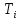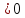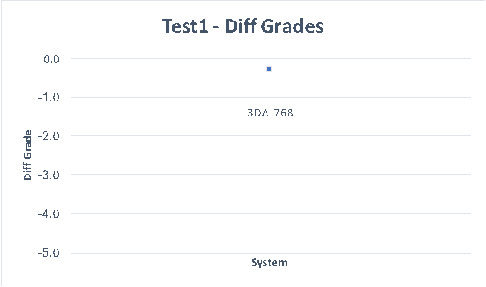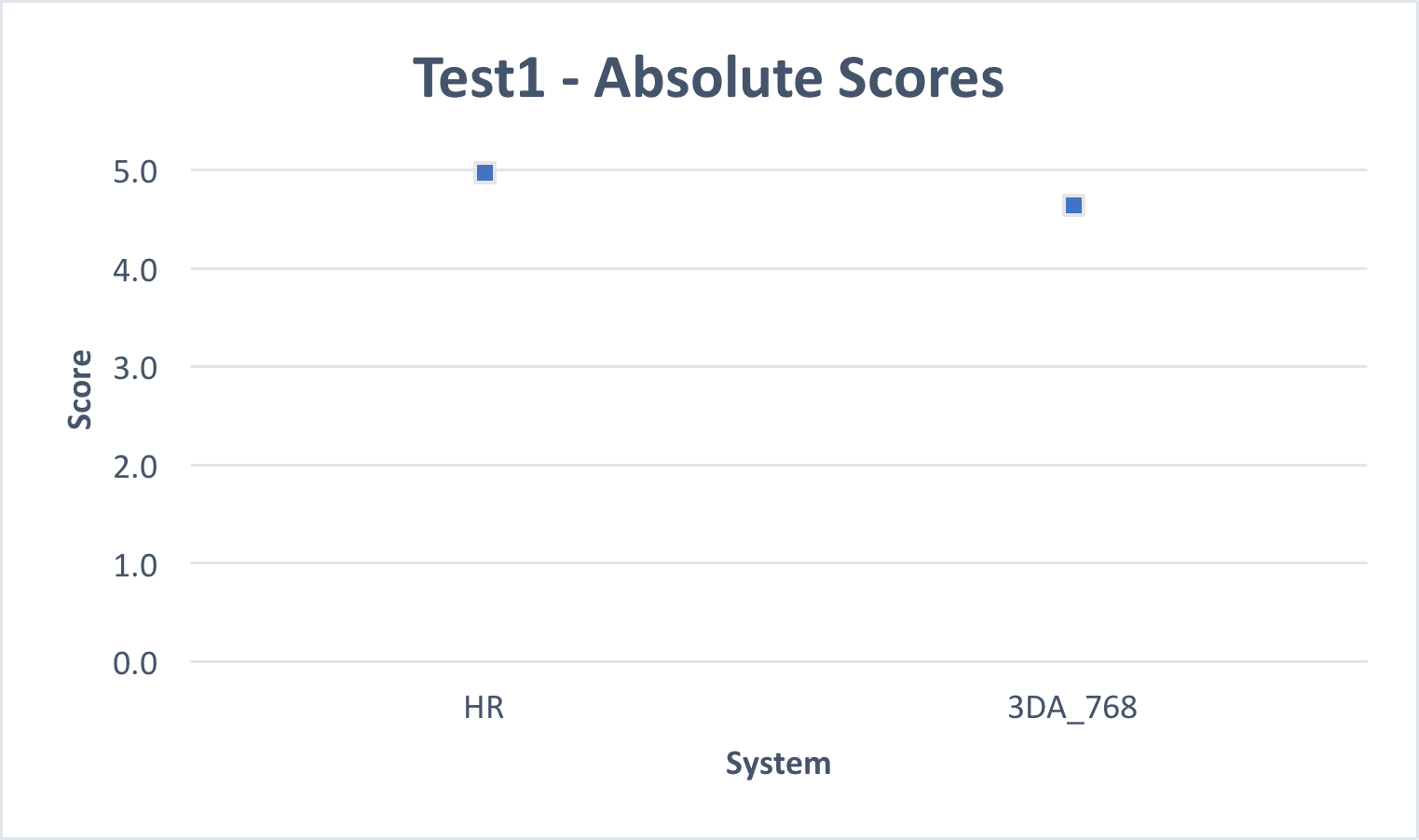# Statistical Analysis and Test Results

 Page 6/14 Date 23.04.2018 Size 1.43 Mb. #46253

1. ## Listener post-screening

Test 1

Test 1 used the BS.1116 test methodology . For each listener in Test 1, post-screening of listener responses was based on the listener’s ability to correctly differentiate the Hidden Reference from the System under Test, which is the procedure recommended in BS.1116-3. The exact procedure used is described in Annex 3.

The post-screening procedures computes the statisticwhich is the 95% point of the cumulative distribution of the listener Diff Grades, which are assumed to have the Student t distribution. Iffor the listener i, then we conclude, with a 95% level of significance, that the listener cannot reliably differentiate between the Hidden Reference and the System under Test, and listener responses for the 12 test items are removed from consideration.
Test 2, Test 3, Test 4

Test 2, Test 3 and Test 4 used the MUSHRA test methodology . For each listener in each test, post-screening of listener responses was based on scores for Hidden Reference and Low Pass filtered anchors. The procedure is as follows:

If, for any test item in a given test, either of the following criterion are not satisfied:

• The listener score for the Hidden Reference is greater than or equal to 90 (i.e. HR >= 90)

• The listener score for the Hidden Reference, the 7.0 kHz lowpass anchor and the 3.5 kHz lowpass anchor are monotonically decreasing (i.e. HR >= LP70 >= LP35).

then all listener responses in that test are removed from consideration.
Post-Screening Result

After applying these listener post-screening rules, the number of listeners remaining for each test is shown in the following table.

 Test Test 1 Test 2 Test 3 Test 4 After Post-Screening 35 43 44 68

After applying post-screening there were at least 35 listeners for every test. This number far exceeds the BS.1116-3 and BS.1534-3 recommendations of at least 20 listeners per test.

1. ## Overview

Statistical analysis was performed on subjective scores remaining after listener post-screening. Details of the statistical analysis are given in Annex 3. For Test 1, a Diff Grade was computed (as Hidden Reference – System under Test scores) and statistics were computed on the Diff Grade. In addition, statistical analysis was performed on absolute scores for Hidden Reference and the System under Test. For Test 2, Test 3 and Test 4, statistics were computed on the absolute MUSHRA scores.
The tables in this section show, for each System under Test (Sys), the mean score (Mean) as averaged over all listeners (after post-screening) and all test items. For each result, the 95% confidence interval on the mean score was computed, and the table shows the upper (High) and lower (Low) limits of the 95% confidence interval.
Note that the 95% confidence interval is shown in every plot, but when retaining the full subjective scale, the interval is obscured by the mark used to indicate the mean value. However, 95% confidence intervals are shown in the tabular presentation of scores.
1. ## Test 1 “Ultra HD Broadcast”

The following table shows the mean score for 3D Audio system operating at 768 kb/s (3DA_768) and the associated high and low 95% confidence interval limits on the mean.

 Sys High Low Mean 3DA_768 -0.27 -0.35 -0.31

The following is a plot of the mean score and 95% confidence interval. The confidence interval is plotted, but is so small that it is within the size of the marker used for the mean.The following table and plot show the mean score for 3D Audio system operating at 768 kb/s (3DA_768), the Hidden Reference (HR) and the associated high and low 95% confidence interval limits on the mean for each condition.
For the 3DA_768, the absolute score is not lower that 4.6 at the 95% level of confidence, which is well above the 4.0 limit recommended in ITU-R BS.1548-4 for “High-quality emission” for broadcast applications (indicated by red line in the plot). Recommendation ITU-R BS.1548-4, Section 2.1.1.1 “High-quality emission” states “Ideally, the quality of the sound reproduced after decoding will be subjectively similar to the original signal for most types of audio programme material. Using the triple stimuli double blind with hidden reference test, described in Recommendation ITU-R BS.1116, this requires mean values consistently higher than 4 on the Recommendation ITU-R BS.1116 5-grade impairment scale at the reference listening position.”

 Sys High Low Mean HR 5.05 4.90 4.98 3DA_768 4.67 4.61 4.64

The following is a plot of the mean scores and 95% confidence intervals. The confidence intervals are plotted, but are so small that they are within the size of the marker used for the mean. The red line shows the ITU-R requirement for “high-quality emission,” i.e. mean value of 4.0.